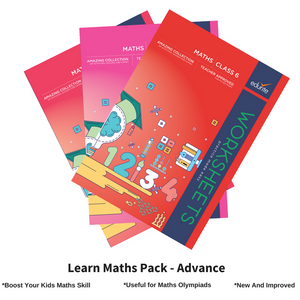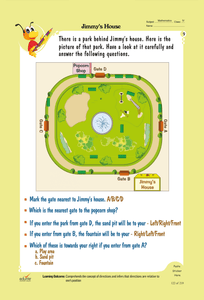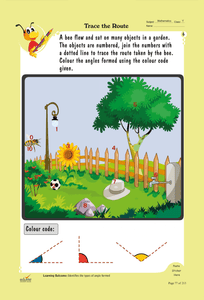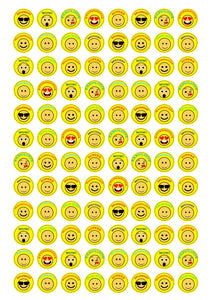# Edurite Learn Maths Pack for Kids Advance (3 Book Combo, Ideal for Ages 8 - 12)

Regular price
Rs. 3,499.00
Sale price
Rs. 2,499.00

Units Sold: 2354

Free Delivery.
Makes Learning Math Fun Again
Successfully Deployed in schools helping more than 4500 children
Rave Reviews from Principals of Schools
Cash on Delivery Available with 7 Day Return Policy

Key features of our worksheets:

• Bright and colorful pages that intrigue students
• Useful for Olympiads and other competitive exams.
• Ideal for Kids who is in class 4 to 6
• Variety of interactive activities that reiterate each concept
• Provide a thorough understanding of the question pattern
• Hard and user-friendly A4 Size pages
• Prepared by the experienced academicians in tune with the updated NCERT syllabus
• Free Study Plan.

Topics covered in Book 1 ( Pages: 219 )

• Solve Problems
• Apply Addition and Discover Number Patterns
• Addition and Subtraction to Solve the Problem
• Find the Average of Two Numbers
• Brick Laying Pattern
• Ways of Writing Dates
• Solve Simple Word Problems
• Solve word problems on week, month and year
• Associate Days with Dates in a Calendar
• Simple Calculations to Find the Age of the Object
• Interpret Data and Solve Problems
• Pie Charts and Bar Graph
• Count the Number of Repeated Words & Letters
• Circle, Semicircle, Diameter and Radius
• Concentric Circles
• Three-dimensional Figure
• Length and Boundary
• Perimeter
• Distances and Measures
• Doubling a Number
• Fractions, Addition, Subtraction
• Measurement of Liquids
• Statement Problems
• Centimeters, Meters and Kilometers
• Money
• Profit and Loss
• Shape Pattern, Designing and Comparing Patterns
• Pascal’s Triangle
• Convert the 12 hour clock to a 24 hour clock
• Multiplication table of 3, 4, 5, 6 and 7
• Multiply by 10, 100
• Division
• Reading the Compass

Topics covered in Book 2 ( Pages: 225)

• Relationship between multiplication and division
• Adding and Subtracting Decimals
• Analyze Statement Problems
• Match the Blocks with their Slots / Jigsaw Puzzles
• Cost and Weight of a given Object
• Interpret the Data based on Colors and Answer Questions
• Identify Objects of Similar Color and Count them
• Demonstrate the Concept of the Decimal Point
• Multiplication and Division Skills
• Verify the Accuracy of the Measurements
• Estimation
• Demonstrate the Concept-Factors and Multiples
• Fractions
• Apply Logical Reasoning Skills and Solve the Problem
• Magic Squares
• Area and perimeter
• Reflection Symmetry
• Palindromes
• Sequence of Letters and Numbers
• Calculate the Volume of a given Shape
• Identify the Types of Angles formed
• Relationship between Angles and their Effects on Objects
• Weight
• Word Problems

Topics covered Book 1 (Pages: 150)

• Greatest & Smallest 4 – digit number - 01
• Ascending order – Descending order
• Shifting Digits
• Place Value 5 - Digit Numbers
• Expanded Form of Numbers
• Reading and Writing Large Numbers
• Estimates to the Nearest Tens, Hundreds, Thousands
• Concept of Estimation
• Estimate Sum or Difference
• Expanding Brackets
• Roman Numerals and its Application
• Properties of Multiplication -01
• Patterns Observation
• Factors and Multiples
• Prime Numbers 1 to 100
• Divisibility Rules
• Factorisation of Number
• HCF & LCM
• Line segment - Types of Lines
• Different types of Polygons
• Angles
• Circles
• Measurement of line
• Angle Measurement Using Protractor
• Triangles & Quadrilaterals
• 2D and 3D Shapes
• Addition of Integers
• Proper, Improper Fractions and Mixed Fractions
• Equivalent Fractions
• Fractions and Decimals
• Comparison of Decimals
• Length & Weight
• Solution of Equation - Word problems
• Ratio & Proportion - Word problems
• Symmetry & Lines of Symmetry
• Perpendicular Lines
• Pictographs
• Bar Graph
• Area of a Square & Rectangle
• Variables

## Customer Reviews

Based on 10 reviews